# Perturbing correlation matrices¶

Sometimes it happens that a correlation matrix falls on us as though from heaven and we have just got to deal with it. There's no underlying data from which it was generated (or at least, this data is not easily available). To make matters worse, the correlation matrix will be used in making critically-important business decisions down the line. (Though this scenario may sound strange / hellish to the uninitiated, readers who work in risk management will be intimately familiar with this unfortunate scenario.)

Short of demanding access to the underlying data, the best case scenario here is for us to at least see what the downstream effects would be of small changes in the underlying correlation structure. In this post we'll explore a few ways to do this using a Bayesian modeling approach.

In :
%matplotlib inline

import torch
import pyro
import pyro.distributions as dist
import matplotlib.pyplot as plt

SEED = 1
torch.manual_seed(SEED)
pyro.set_rng_seed(SEED)


First we'll generate a correlation matrix from some arbitrary covariance matrix. We draw $L \sim \text{MultivariateNormal}(0, I)$ on $\mathbb{R}^{D(D+1)/2}$, reshape it into a lower-triangular matrix in $\mathbb{R}^{D\times D}$, construct the covariance matrix as $\Sigma = L L^\dagger$, and then finally have our correlation matrix $C = \sigma^{-1} \Sigma \sigma^{-1}$, where $\sigma = \sqrt{\text{diag }\Sigma}$.

In :
dim = 10
chol_vec = torch.randn(
(int(0.5 * dim * (dim + 1)),)
)
chol_mat = torch.zeros((dim, dim))
inds = torch.tril_indices(row=dim, col=dim, offset=0)
chol_mat[
inds, inds
] = chol_vec
cov = torch.matmul(chol_mat, chol_mat.T)

In :
# covariance matrix
im = plt.imshow(cov)
cbar = plt.colorbar(im)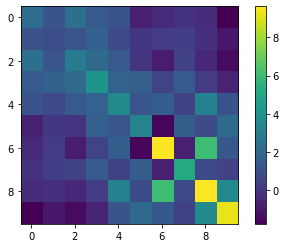In :
# correlation matrix
sqrt_diag = torch.diag(torch.diag(cov)).sqrt()
inv_sqrt_diag = torch.pinverse(sqrt_diag)
corr = inv_sqrt_diag.matmul(cov).matmul(inv_sqrt_diag)

im = plt.imshow(corr)
cbar = plt.colorbar(im)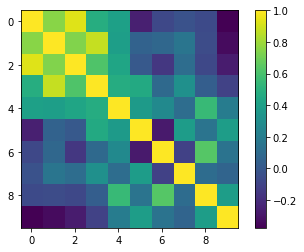Okay, fine. We have tasked ourselves with finding correlation matrices that are "close" to this one in some way. We'll propose and estimate two different Bayesian models for doing this, each of which have pros and cons.

## Normed LKJ model¶

A very simple generative model for correlation matrices is given by \begin{aligned} \eta &\sim p(\eta),\\ C &\sim \text{LKJ}(C|\eta). \end{aligned}

The Lewandowski-Kurowicka-Joe (LKJ) distribution is a flexible distribution defined over correlation matrices. It has the density $p(C|\eta) \propto (\det\ C)^{\eta - 1}$ --- a great reference on this distribution is the Stan documentation. This distribution doesn't have a location or scale parameter, so our strategy for inferring matrices that are close to the observed correlation matrix will be bipartite. First we'll infer the (approximate variational) posterior distribution of $\eta$, $q_{\psi}(\eta)$, and draw samples from the posterior predictive distribution, $C' \sim p(C'|C) = \int d\eta\ \text{LKJ}(C'|\eta)\ q_{\psi}(\eta)$. Then we'll sort the draws from the posterior predictive based on some distance from the observed correlation matrix.

In :
def corr_model(corr=None, hyper_eta=1., dim=10):
eta = pyro.sample(
'eta',
dist.Gamma(hyper_eta, 1.)  # E[eta] = hyper_eta
)
lkj = pyro.sample(
'lkj',
dist.LKJCorrCholesky(dim, eta=eta),
obs=corr
)
return lkj


We'll fit the posterior by maximizing the ELBO. (Read the Pyro docs if you don't know what this is.) For numerical stability, the LKJ distribution in Pyro is actually defined over Cholesky factors of the correlation matrix instead of the correlation matrix itself. We'll use a diagonal normal distribution as the guide --- since there's only a single, univariate latent rv, we aren't missing out on correlations in the posterior by doing this (though the transformed normal density still might not be close to the shape of the true posterior). Under the hood, Pyro approximates the true posterior defined over $(0, \infty)$ with a normal distribution by transforming $\eta$ into a latent unconstrained space.

In :
model = lambda x: corr_model(corr=x, hyper_eta=1., dim=dim)
guide = pyro.infer.autoguide.AutoDiagonalNormal(model)
svi = pyro.infer.SVI(model, guide, optim, loss=pyro.infer.Trace_ELBO())

pyro.clear_param_store()
choled_corr = corr.cholesky()
losses = []

for n in range(2000):
loss = svi.step(choled_corr)
losses.append(loss)
if n % 500 == 0:
print(f"On iter {n}, loss = {loss}")

for name, value in pyro.get_param_store().items():
print(name, pyro.param(name))  # log transformed to unconstrained parameter space

plt.plot(losses)
plt.xlabel('Iterations')
plt.ylabel('Trace ELBO')

On iter 0, loss = 48.7463036775589
On iter 500, loss = 34.43329858779907
On iter 1000, loss = 34.07880884408951
On iter 1500, loss = 34.24194511771202
AutoDiagonalNormal.loc Parameter containing:

Out:
Text(0, 0.5, 'Trace ELBO')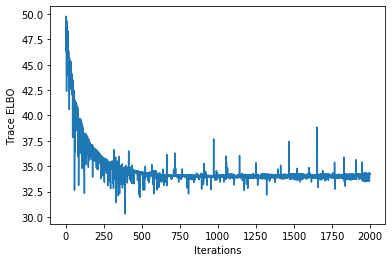Great. The loss plot looks converge-y, though there's still a fair amount of fluctuation from iteration to iteration. Let's see what a few of these correlation matrices look like. Remember that we actually fit Cholesky factors, so we have to batch multiply these to create the correlation matrices.

In :
predictive = pyro.infer.Predictive(
model,
guide=guide,
num_samples=10000,
return_sites=['lkj']
)
chol_matrices = predictive(None)['lkj']
corr_matrices = torch.einsum(
'aij, akj -> aik',  # batch matrix multiplication with transpose
chol_matrices,
chol_matrices
)

inds = torch.randint(corr_matrices.shape, (8,))
fig, axes = plt.subplots(3, 3, figsize=(6, 6))

for ind, ax in zip(inds, axes.flatten()):
im_ind = ax.imshow(corr_matrices[ind], vmin=-1, vmax=1)

true_im = axes[-1, -1].imshow(corr, vmin=-1, vmax=1,)
axes[-1, -1].set_title('true corr')
for a in ['top','bottom','left','right']:
axes[-1, -1].spines[a].set_linewidth(2.)

cbar_ax = fig.add_axes([0.85, 0.15, 0.05, 0.7])
fig.colorbar(im, cax=cbar_ax)

Out:
<matplotlib.colorbar.Colorbar at 0x12ad30710>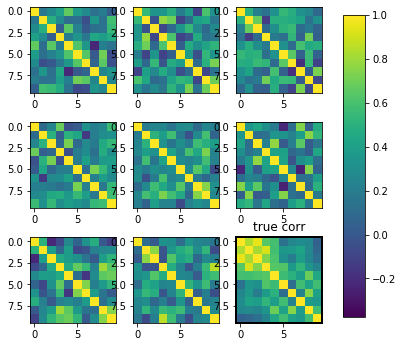Huh.

Now let's see what the closest draws from this distribution to the observed correlation matrix look like. We will use two different norms --- the $\ell_2$ (i.e. Frobenius) and the $\ell_\infty$ --- even though they are mathematically equivalent. In the context of matrices, we'll define these norms as

$$||C - C'||_2^2 = \frac{1}{D^2}\sum_{d,d'}(C_{d,d'} - C'_{d,d'})^2$$

and $$||C - C'||_{\infty} = \max_{d}\max_{d'} |C_{d,d'} - C'_{d,d'}|.$$

The constants out front really don't matter#Properties).

In :
l2_mats = sorted(
zip(
corr_matrices,
corr_matrices.sub(corr).pow(2.).mean(dim=(-2, -1))
),
key=lambda t: t[-1]
)
linfty_mats = sorted(
zip(
corr_matrices,
corr_matrices.sub(corr).abs().max(dim=-2).max(dim=-1)
),
key=lambda t: t[-1]
)

In :
fig, axes = plt.subplots(3, 3, figsize=(6, 6))

for (mat, norm), ax in zip(l2_mats[:8], axes.flatten()):
im_ind = ax.imshow(mat, vmin=-1, vmax=1)
ax.set_title(f'$\ell_2$ norm = {round(float(norm.numpy()), 4)}')

true_im = axes[-1, -1].imshow(corr, vmin=-1, vmax=1,)
axes[-1, -1].set_title('true corr')
for a in ['top','bottom','left','right']:
axes[-1, -1].spines[a].set_linewidth(2.)

plt.tight_layout()

cbar_ax = fig.add_axes([0.85, 0.15, 0.05, 0.7])
fig.colorbar(im, cax=cbar_ax)

Out:
<matplotlib.colorbar.Colorbar at 0x12c350630>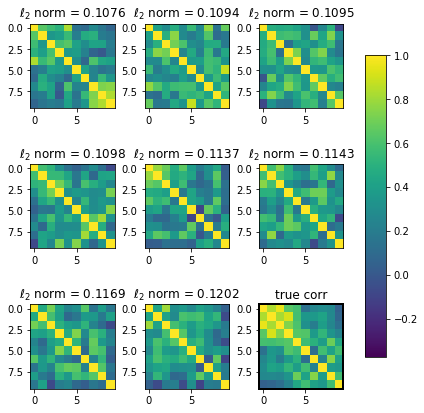In :
fig, axes = plt.subplots(3, 3, figsize=(6, 6))

for (mat, norm), ax in zip(linfty_mats[:8], axes.flatten()):
im_ind = ax.imshow(mat, vmin=-1, vmax=1)
ax.set_title(f'$\ell_\infty$ norm = {round(float(norm.numpy()), 4)}')

true_im = axes[-1, -1].imshow(corr, vmin=-1, vmax=1,)
axes[-1, -1].set_title('true corr')
for a in ['top','bottom','left','right']:
axes[-1, -1].spines[a].set_linewidth(2.)

plt.tight_layout()

cbar_ax = fig.add_axes([0.85, 0.15, 0.05, 0.7])
fig.colorbar(im, cax=cbar_ax)

Out:
<matplotlib.colorbar.Colorbar at 0x12c6a3748>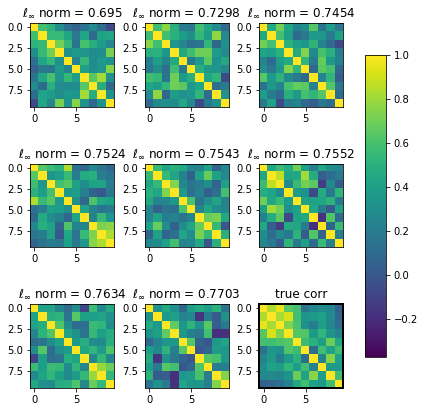Under both norms, these matrices seem to exhibit blocked structures similar to the original correlation matrix, which is a nice property. However, the mean of the LKJ distribution is the identity matrix, so in this sense it isn't a vary good quantitative representation of the original correlation matrix.

## Transformed normal model¶

Instead of considering correlation matrices in their entirety, we'll now just think about flattened vectors $x \in [-1, 1]^{D(D - 1)/2}$ that represent the offset-by-one lower triangular factor of a correlation matrix. We'll model this vector with a multivariate normal distribution in transformed unconstrained space, $y \in \mathbb{R}^{D(D-1)/2}$,

$$p(y|\mu, \Sigma) = \left( \frac{1}{2\pi (\det \Sigma)^{1/D}} \right)^{D/2} \exp\left[ -\frac{1}{2}(y - \mu)^\dagger \Sigma^{-1} (y - \mu) \right].$$

Mapping $x = \tanh y$ gives a arctanh-Normal distribution,

\begin{align} p(x|\mu, \Sigma) &= p(\tanh^{-1}(x)|\mu, \Sigma)\ \det|J(\tanh^{-1}(x))|\\ &= \mathcal N \exp\left[ -\frac{1}{2}\left(\frac{1}{2}\log \frac{x + 1}{x - 1} - \mu\right)^\dagger \Sigma^{-1} \left(\frac{1}{2}\log \frac{x + 1}{x - 1} - \mu\right) \right] \end{align},

with the normalization constant given by $$\mathcal{N}^{-1} = \left( \frac{1}{2\pi (\det \Sigma)^{1/D}} \right)^{D/2} \prod_{d=1}^D \frac{1}{1 - x_d^2}.$$

We'll denote this distribution simply by $x \sim \text{arctanh-Normal}(\mu, \Sigma)$. The generative model is now

\begin{aligned} \Sigma &\sim p(\Sigma)\\ \mu &\sim p(\mu)\\ C &\sim \text{arctanh-Normal}(C|\mu, \Sigma). \end{aligned}

Though we could code the above likelihood function, when there's no observation error in the correlation matrix (as we're assuming in our example) it'll just be easier to backtransform the upper triangular vector into the latent unconstrained space, fit a multivariate normal model there, then forward-transform the posterior predictive draws back into the constrained space.

In :
tril_inds = torch.tril_indices(row=dim, col=dim, offset=-1)
tril_corr_vec = corr[
tril_inds, tril_inds
]

def arctanh(x):
return 0.5 * torch.log(
(1. + x) / (1. - x)
)

norm_corr_vec = arctanh(tril_corr_vec)
new_dim = int(0.5 * dim * (dim - 1))

def tanh_normal_corr_model(vec=None,):
hyper_var = pyro.sample(
'hyper_var',
dist.LogNormal(0., 1.).expand((new_dim,))
)
hyper_cov = torch.diag(hyper_var,)

hyper_mu = pyro.sample(
'hyper_mu',
dist.MultivariateNormal(
norm_corr_vec,
covariance_matrix=0.1 * torch.eye(new_dim,)
)
)

latent_normal = pyro.sample(
'latent_normal',
dist.MultivariateNormal(
hyper_mu,
covariance_matrix=hyper_cov,
),
obs=vec
)
return latent_normal


This time we'll perform inference using Hamiltonian Monte Carlo.

In :
kernel = pyro.infer.NUTS(tanh_normal_corr_model)
mcmc = pyro.infer.MCMC(
kernel=kernel,
num_samples=2000,
warmup_steps=250,
)
posterior = mcmc.run(norm_corr_vec)

Sample: 100%|██████████| 2250/2250 [01:19, 28.20it/s, step size=4.10e-01, acc. prob=0.877]

In :
posterior_predictive = pyro.infer.Predictive(
tanh_normal_corr_model,
posterior,
num_samples=1000
)
ppc = posterior_predictive()['latent_normal']

latent_corrs = torch.zeros((ppc.shape, dim, dim))

for i, (sub, vec) in enumerate(zip(latent_corrs, ppc)):
sub[tril_inds, tril_inds] = torch.tanh(vec)
sub = sub.T
sub[tril_inds, tril_inds] = torch.tanh(vec)
sub += torch.eye(dim)


Let's compare the posterior predictive mean correlation matrix with the observed matrix.

In :
fig, axes = plt.subplots(1, 3, figsize=(10, 5))
ax, ax2, ax3 = axes

im1 = ax.imshow(corr, vmin=-1, vmax=1)
im2 = ax2.imshow(latent_corrs.mean(dim=0), vmin=-1, vmax=1)
im3 = ax3.imshow(latent_corrs.std(dim=0), vmin=-1, vmax=1)

cbar_ax = fig.add_axes([0.85, 0.15, 0.05, 0.7])
fig.colorbar(im, cax=cbar_ax)

Out:
<matplotlib.colorbar.Colorbar at 0x12db42c50>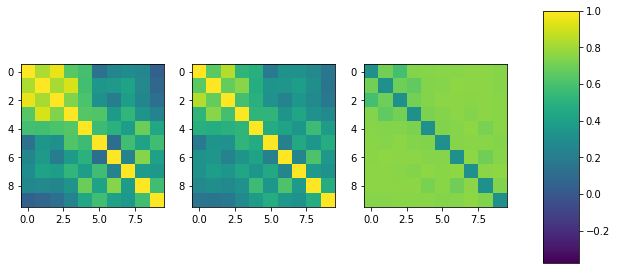Ah, good. We've achieved our objective --- the posterior mean of this distribution is essentially a regularized version of the observed correelation matrix.

What do random draws from this distribution look like?

In :
inds = torch.randint(latent_corrs.shape, (8,))
fig, axes = plt.subplots(3, 3, figsize=(6, 6))

for ind, ax in zip(inds, axes.flatten()):
im_ind = ax.imshow(latent_corrs[ind], vmin=-1, vmax=1)

true_im = axes[-1, -1].imshow(corr, vmin=-1, vmax=1,)
axes[-1, -1].set_title('true corr')
for a in ['top','bottom','left','right']:
axes[-1, -1].spines[a].set_linewidth(2.)

cbar_ax = fig.add_axes([0.85, 0.15, 0.05, 0.7])
fig.colorbar(im, cax=cbar_ax)

Out:
<matplotlib.colorbar.Colorbar at 0x12df75b70>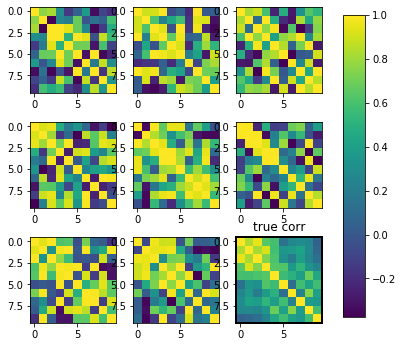Ooh. It looks like we've given up the nice regular block structure of the "close" posterior predictive draws from the LKJ distribution. These draws are all over the place --- but, as we noted above, they average out to be "close" to the observed matrix. We could certainly improve on the quality of this posterior by including a more informative distribution over the hyper covariance instead of just an expanded univariate LogNormal.

## Consequences¶

We went through this exercise for a reason: we want to know what perturbations of various kinds would do to a downstream model. Let's look at a very simple model,

$$z_{t + 1} = z_t + L_nw_t,\ t=0,...,N_t-1$$

where $L_n$ is the Cholesky decomposition of $\Sigma_n$, $\Sigma_n = \sqrt{\text{diag }\Sigma} C_n \sqrt{\text{diag }\Sigma}$, and $w_t \sim \text{MultivariateNormal}(0, I)$. We'll run each simulation with the same random seed so that the same sequence of white noise is driving the system; the only differences in the observed time series are due to the different correlation matrices.

In :
def paths(corr, Nt=10000, eps=1e-5, SEED=1):
torch.manual_seed(SEED)
pyro.set_rng_seed(SEED)

try:
torch.zeros(corr.shape[-1]), covariance_matrix=sqrt_diag.matmul(corr).matmul(sqrt_diag)
).sample((Nt,)).cumsum(dim=0)

except RuntimeError:  # correlation matrix is not positive definite due to numerical issues
corr += eps * torch.eye(dim)  # augment with a very small multiple of identity matrix
corr /= (1. + eps)  # the diagonal is now all ones again
torch.zeros(corr.shape[-1]), covariance_matrix=sqrt_diag.matmul(corr).matmul(sqrt_diag)
).sample((Nt,)).cumsum(dim=0)

mean_paths = paths(latent_corrs.mean(dim=0))
l2_paths = paths(l2_mats)
linfty_paths = paths(linfty_mats)
true_paths = paths(corr)

In :
fig, axes = plt.subplots(int(dim / 2), 2, figsize=(8, 8))
axes_ = axes.flatten()
for x, y, z, a, ax in zip(mean_paths.T, true_paths.T, linfty_paths.T, l2_paths.T, axes_):
ax.plot(x, color='k', linewidth=0.5)
ax.plot(y, color='royalblue', linewidth=0.5)
ax.plot(z, color='crimson', linewidth=0.5)
ax.plot(a, color='goldenrod', linewidth=0.5)

# for labels only
axes[-1][-1].plot(x, color='k', linewidth=0.5, label='arctanh-Normal')
axes[-1][-1].plot(y, color='royalblue', linewidth=0.5, label='Observed')
axes[-1][-1].plot(z, color='crimson', linewidth=0.5, label='$\ell_2$-close LKJ')
axes[-1][-1].plot(a, color='goldenrod', linewidth=0.5, label='$\ell_{\infty}$-close LKJ')
axes[-1][-1].legend(framealpha=0., bbox_to_anchor=(1.1, 1.05))

for ax in axes:
ax.set_xticks([])
ax.set_xticklabels([])

for ax in axes[-1]:
ax.set_xlabel('$t$ (unitless time)')

plt.tight_layout()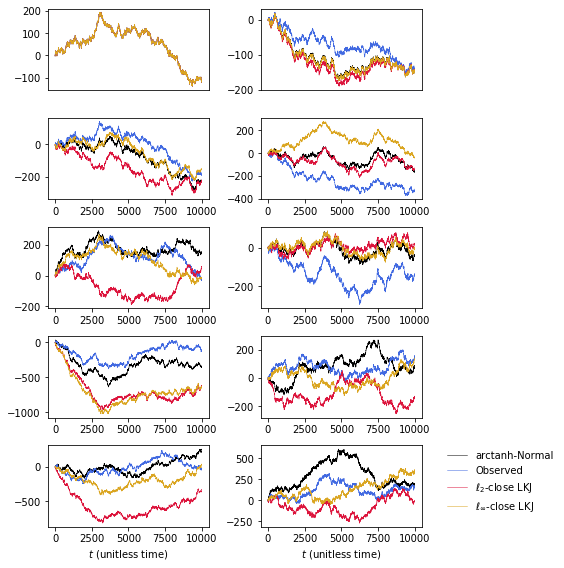The $d$-th panel displays the $d$-th dimension of the $D$-dimensional random walk. Across each dimension, realizations of the random walk are obviously correlated but display substantial variation in final values.

Hopefully you can think of applications of these perturbation models --- for example, to analysis of risk allocations under value-at-risk or expected shortfall.